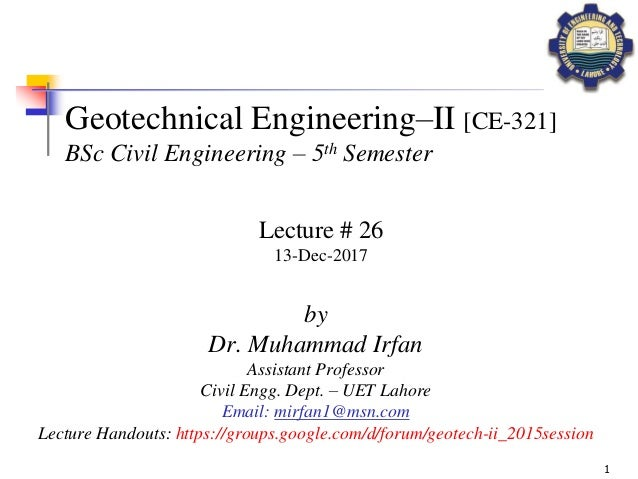Successfully reported this slideshow.Upcoming SlideShare
×

# Geotechnical Engineering-II [Lec #26: Slope Stability]

Class notes of Geotechnical Engineering course I used to teach at UET Lahore. Feel free to download the slide show.
Anyone looking to modify these files and use them for their own teaching purposes can contact me directly to get hold of editable version.

• Full Name
Comment goes here.

Are you sure you want to Yes No### Geotechnical Engineering-II [Lec #26: Slope Stability]

1. 1. 1 Geotechnical Engineering–II [CE-321] BSc Civil Engineering – 5th Semester by Dr. Muhammad Irfan Assistant Professor Civil Engg. Dept. – UET Lahore Email: mirfan1@msn.com Lecture Handouts: https://groups.google.com/d/forum/geotech-ii_2015session Lecture # 26 13-Dec-2017
2. 2. 2 STABILITY OF SLOPES Slope An exposed ground surface that stands at an angle with the horizontal is called an unrestrained slope.
3. 3. 3 TYPES OF SLOPES A) w.r.t. Method of Construction 1. Natural Slopes 2. Man-made / Engineered Slopes Embankments, earthen dams, river dikes, excavation trenches, etc. Engineered SlopeNatural Slope
4. 4. 4 TYPES OF SLOPES B) w.r.t. Extents 1. Infinite Slopes Having constant slope of infinite extent, e.g. long slope of a mountain face. 2. Finite Slopes Slopes of limited heights and extents, e.g. typical man-made slopes Finite SlopeInfinite Slope
5. 5. 5 TYPES OF SLOPES C) w.r.t. Slope Material 1. Cohesionless 2. Cohesive Cohesionless Cohesive
6. 6. 6 STABILITY OF SLOPES A slope is said to be stable if it meets a prescribed need for a fixed period of time with a suitable safety factor (FOS). Nova Frebergo, Brazil January 13, 2011 California, USA January, 1997
7. 7. 7 CAUSES OF SLOPE FAILURE
8. 8. 8 CAUSES OF SLOPE FAILURE
9. 9. 9 CAUSES OF SLOPE FAILURE
10. 10. 11 TYPES/MODES OF SLOPE FAILURE Toe Failure  Failure surface passing through toe of slope  Material of slope is homogeneous  Relatively steep slope angles
11. 11. 12 TYPES/MODES OF SLOPE FAILURE Base Failure  Failure surface passing through foundation  Foundation soil somehow weaker than slope material  Relatively gentle slopes
12. 12. 13 SLOPE STABILIZING MEASURES
13. 13. 14 SLOPE STABILIZING MEASURES
14. 14. 15 SLOPE STABILIZING MEASURES
15. 15. 16 LIMIT EQUILIBRIUM ANALYSIS  Most common quantitative measure of slope stability  Stability of slope expressed in terms of factor of safety just before failure 𝐹𝑂𝑆 = 𝑅𝑒𝑠𝑖𝑠𝑡𝑖𝑛𝑔 𝐹𝑜𝑟𝑐𝑒 𝐷𝑖𝑠𝑡𝑢𝑟𝑏𝑖𝑛𝑔 𝐹𝑜𝑟𝑐𝑒 𝐹𝑂𝑆 = 𝐴𝑣𝑎𝑖𝑙𝑎𝑏𝑙𝑒 𝑆ℎ𝑒𝑎𝑟 𝑆𝑡𝑟𝑒𝑛𝑔𝑡ℎ 𝑜𝑓 𝑆𝑜𝑖𝑙 𝐴𝑝𝑝𝑙𝑖𝑒𝑑 𝑆ℎ𝑒𝑎𝑟 𝑆𝑡𝑟𝑒𝑠𝑠 Failure Surface FOS < 1 FOS = 1 FOS > 1 Typical design criterion for stable slope; FOS > 1.5 → Failure → Verge of failure/Just stable → Stable
16. 16. 17 SLOPE STABILITY ANALYSIS Infinite Slope Assumptions: 1. Slope face is planar and of infinite extent 2. Failure surface is║to slope face 3. Water surface is║to slope face 𝐹𝑂𝑆 = 𝐴𝑣𝑎𝑖𝑙𝑎𝑏𝑙𝑒 𝑆ℎ𝑒𝑎𝑟 𝑆𝑡𝑟𝑒𝑛𝑔𝑡ℎ 𝑜𝑓 𝑆𝑜𝑖𝑙 𝐴𝑝𝑝𝑙𝑖𝑒𝑑 𝑆ℎ𝑒𝑎𝑟 𝑆𝑡𝑟𝑒𝑠𝑠 L H h a a b c d
17. 17. 18 SLOPE STABILITY ANALYSIS Infinite Slope Case-A: c-f Soil (c>0; f>0) L H h a W a b c d T N a Available Shear Strength of Soil (tr) tr 𝜏 𝑟 = 𝑐′ + 𝜎 𝑛′ ∙ tan 𝜑′ Normal stress on the failure plane 𝜎 𝑛 = 𝑁 ( 𝐿 cos 𝛼)(1) = 𝛾𝐻𝐿 cos 𝛼 ( 𝐿 cos 𝛼)(1) = 𝛾𝐻𝑐𝑜𝑠2 𝛼 𝑊 = 𝛾𝐻𝐿 𝑁 = 𝑊 cos 𝛼 = 𝛾𝐻𝐿 cos 𝛼 𝑇 = 𝑊 sin 𝛼 = 𝛾𝐻𝐿 sin 𝛼 𝑭𝑶𝑺 = 𝑨𝒗𝒂𝒊𝒍𝒂𝒃𝒍𝒆 𝑺𝒉𝒆𝒂𝒓 𝑺𝒕𝒓𝒆𝒏𝒈𝒕𝒉 𝒐𝒇 𝑺𝒐𝒊𝒍 𝑨𝒑𝒑𝒍𝒊𝒆𝒅 𝑺𝒉𝒆𝒂𝒓 𝑺𝒕𝒓𝒆𝒔𝒔
18. 18. 19 SLOPE STABILITY ANALYSIS Infinite Slope Applied Shear Stress (t) 𝜏 = 𝑇 ( 𝐿 cos 𝛼)(1) 𝜏 = 𝛾𝐻𝐿 sin 𝛼 ( 𝐿 cos 𝛼)(1) 𝜏 = 𝛾𝐻 sin 𝛼 cos 𝛼 𝐹𝑂𝑆 = 𝑐′ + 𝛾𝐻𝑐𝑜𝑠2 𝛼 ∙ tan 𝜙′ 𝛾𝐻 sin 𝛼 cos 𝛼 Factor of Safety (FOS) Case-A: c-f Soil (c>0; f>0) L H h a W a b c d T N a tr 𝑭𝑶𝑺 = 𝑨𝒗𝒂𝒊𝒍𝒂𝒃𝒍𝒆 𝑺𝒉𝒆𝒂𝒓 𝑺𝒕𝒓𝒆𝒏𝒈𝒕𝒉 𝒐𝒇 𝑺𝒐𝒊𝒍 𝑨𝒑𝒑𝒍𝒊𝒆𝒅 𝑺𝒉𝒆𝒂𝒓 𝑺𝒕𝒓𝒆𝒔𝒔 Available Shear Strength of Soil (tr) 𝜏 𝑟 = 𝑐′ + 𝛾𝐻𝑐𝑜𝑠2 𝛼 ∙ tan 𝜙′ Without considering the effect of WT
19. 19. 20 SLOPE STABILITY ANALYSIS Infinite Slope 𝐹𝑂𝑆 = 𝑐′ + 𝜎 𝑛′ ∙ tan 𝜙′ 𝛾𝐻 sin 𝛼 cos 𝛼 Case-A: c-f Soil (c>0; f>0)  c’ & f’; effective strength parameters  Obtained through drained triaxial test 𝜎 𝑛 = 𝜎 𝑛 ′ = 𝐴𝐵 = ℎ 𝐴𝐶 = L H h a W a b c d T N a tr A C B D a 𝐴𝐷 = ℎ 𝑤 = 𝑢 = ℎ 𝑤 = 𝑢 = 𝛾 𝑤ℎ 𝑐𝑜𝑠2 𝛼 𝐹𝑂𝑆 = 𝑐′ + (𝛾𝐻 − 𝛾 𝑤ℎ) ∙ 𝑐𝑜𝑠2 𝛼 tan 𝜙′ 𝛾𝐻 sin 𝛼 cos 𝛼 𝛾𝐻𝑐𝑜𝑠2 𝛼 𝜎 𝑛 − 𝑢 ℎ cos 𝛼 𝐴𝐶 cos 𝛼 = ℎ 𝑐𝑜𝑠2 𝛼 𝛾 𝑤ℎ 𝑤 𝐴𝐷 = ℎ 𝑐𝑜𝑠2 𝛼 Pore water pressure, u Considering presence of WT
20. 20. 21 CONCLUDED REFERENCE MATERIAL Principles of Geotechnical Engineering – (7th Edition) Braja M. Das Chapter #13 Essentials of Soil Mechanics and Foundations (7th Edition) David F. McCarthy Chapter #17 Geotechnical Engineering – Principles and Practices – (2nd Edition) Coduto, Yueng, and Kitch Chapter #17